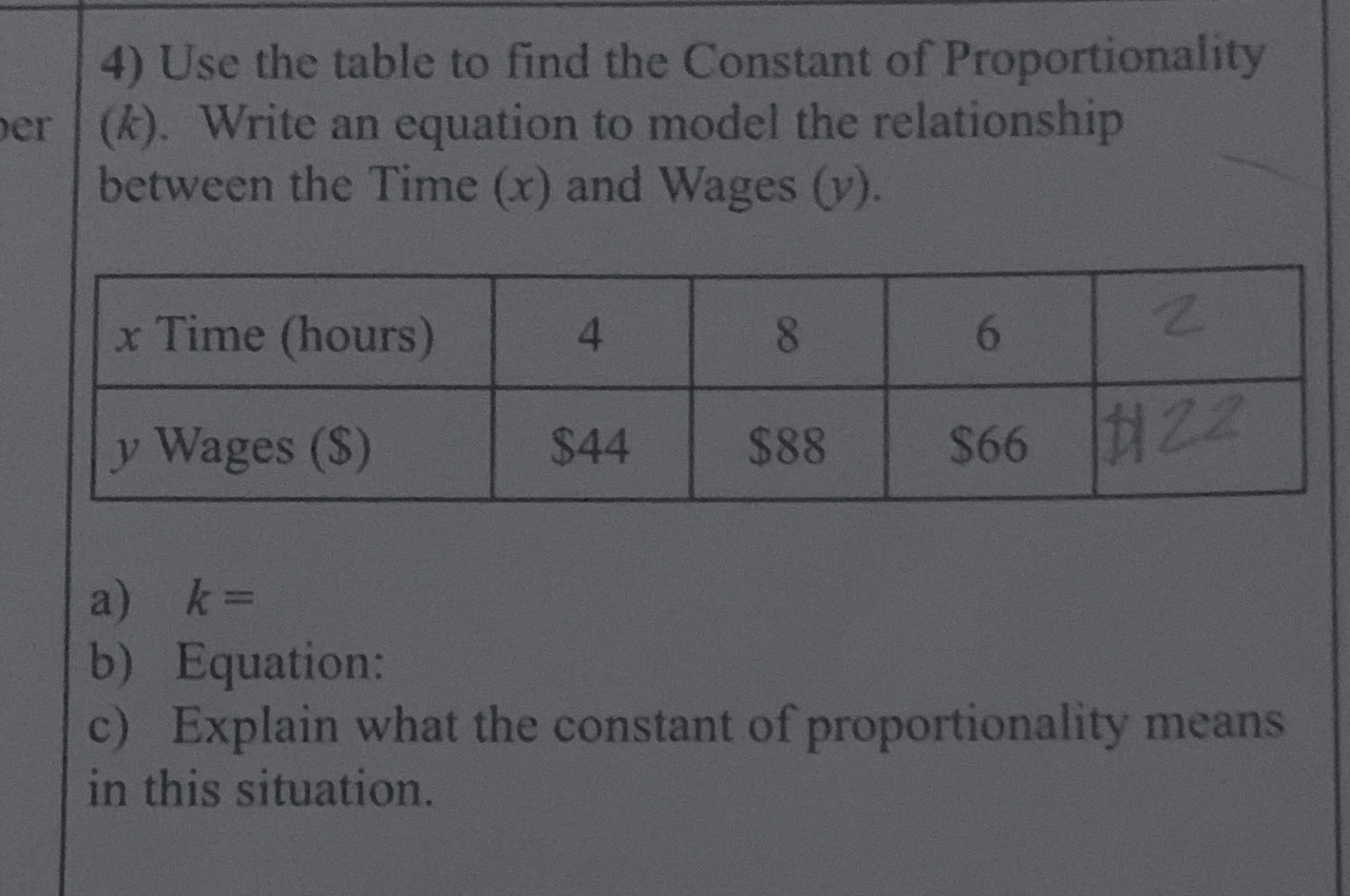### ¿Todavía tienes preguntas de matemáticas?

Pregunte a nuestros tutores expertos
Algebra
Pregunta4) Use the table to find the Constant of Proportionality $$( k )$$ . Write an equation to model the relationship between the Time $$( x )$$ and Wages $$( y )$$ .

a) $$k =$$

b) Equation:

c) Explain what the constant of proportionality means in this situation.